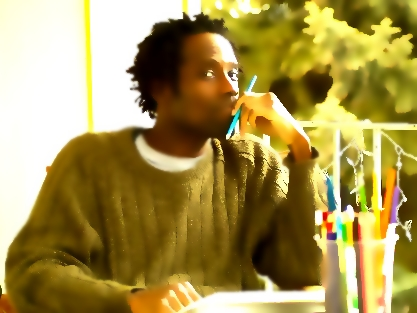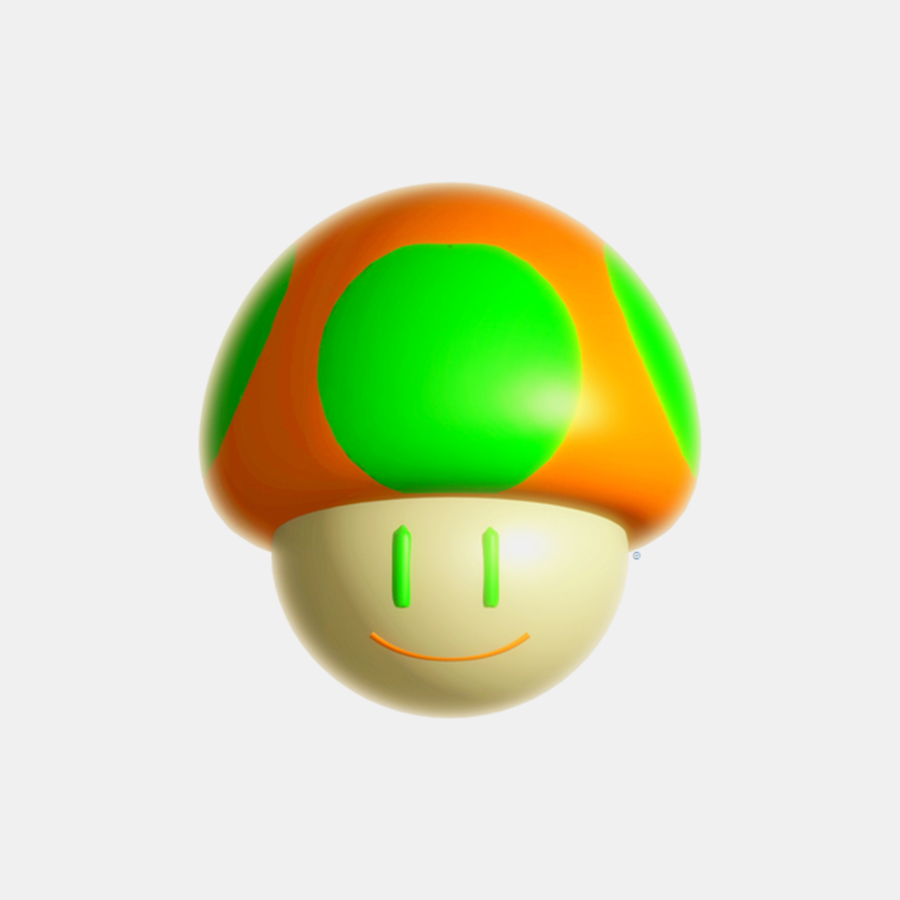Showing posts with label lowtech. Show all posts
Showing posts with label lowtech. Show all posts

### +The Egglepple Company

The Egglepple Company (or just Egglepple) is the pseudonym given to what is now known as Egglepple (kindergarten). It was an incorporated@ business entity in various states throughout the United States of America prior to its deprecation, reorganization, and later inclusion into lnq.@@ Reference: Ohio Secretary of State's Office

Historically, Egglepple was the very first establishment to be a standalone/independent recreational mathematics+ enterprise (+:=contract-based, sometimes ghostwriting for academe from 10/2000 - 02/2001 (also of interest The Stewart Ellis Company - Gobotech, Link Starbury Network, #305)/// pm:=pure mathematics, am:=applied mathematics. (see Funshine/Toonlight)

"Egglepple" is the middle name of 🧑🏿Link Egglepple Starbureiy, after whom the company is called, and by whom the entity was created. It spawned from the unincorporated/unorganized Egglepple portfolio (for toy [🧩puzzle] design, circa 1991).
/// Nowadays, the 'company' portion is assumed to be my roster (of characters/personalities +gallery) and the yots.Back at The Ohio State University, I put in my time roving the halls of the `mathematics`, physics/astronomy, and chemistry departments. I was fairly new in that world ("publish or perish") and really wasn't familiar with LaTeX and that publishing scheme back then (the departments certainly didn't make it easy for non-staff to have access to the system), so I did what I did best when it came to visual expression, I drew stuff up/wrote stuff down on napkins (yes, at times with wax crayons🖍️ or colored pencils✏️). The astronomy department was awesome in the regard that they treated people who came through their doors respectfully. The `math` department, however, was the total opposite. There was so much ego (mine included) polluting the building that it was almost laughable, yet frustrating. [-- `Mathematicians` can be a pompous bunch who struggle for trivial `results`, and their demeanor reflects that. --] Anyway, there would be these 'challenge' `problems`/help requests from the community (ie. intra-departmental) often posted on bulletins, offering modest bounties💵 (very short-term `contracts`) for their `solutions`. The opportunist in me would peel these from the boards, take them home, and come back shortly thereafter with my answer(s) written on (paperclipped🖇️) napkins. I remember just vagabonding off of those purses (yes, I was a 'bounty hunter') for periods at a time (sometimes in conjunction with all-levels `math` tutoring/coaching gigs). I guess that - in addition to responding to Michio Kaku's earlier proposition - was me (being the person responsible for) turning `math` into my version of a sport (, and thus, solidifying my interest in ctfs🏁).

### +mathemusic

Mathemusic is the functional conflation of brane classes defined as 'an incorporation of keys and timing to perturb a sinusoidal, whose resultant is a γ-proof'.

This tends more towards being a supermathematical exercise grounded in the principles of music theory. However, it is practically expressing (with libretti) statistics in the framework of 🎵music (even sans sonically). Cryptologically, it is the algorithmic direction of variation*.** `Solutions` have `melodic` texture.

When we speak of mathemusic, we are generally doing so in the context of The Origamic Symphony🎶/Egglepple. (see ¢ent, mathletics, 🧑🏿lnq's Starting Five, 3-rex, fibor, UUe, juke notation, QQ)

"All I hear is mathematics. All I see is music." - 🧑🏿Link Starbureiy/// Contemporary mathemusic was originally formulated by 🧑🏿Link Starbureiy.🤓

### +mathematics

Mathematics is an ambiguous way to prove or disprove ideal behaviors of objects.
/// An '`open` story of counting', it must be said that mathematical methodology is a cumulative effort (ie. rigorously `built` atop previous checks).

As libraries* for computing functions, mathematicians use its models to formulate theories/theorems/identities/errata.** We acknowledge the so-called 'Big Five (5)' active areas in mathematics to be [1-5 labeled alphabetically]: algebra , analysis , arithmetic , geometry , and music . As far as I am concerned, the basis of math is `juking`. (see also mathemusic, mathletics, recreational mathematics, mathematical model, Mathilda, 📓So, you want to be a mathematician?, The Mathemagician, Opus Solve, physics, information science, game, cryptosport, 🧩puzzle)

"The essence of mathematics is not proof, but conjecture." - 🧑🏿Link Starbureiy

/// +There are three (3) `classes` of mathematics: pure, applied, and recreational (pure,applied ∈ recreational). This `definition` covers all three (3), and may be referred to as polymathematics. A mathematician is someone who advances `classical` mathematics.
+In my line of work, I think of so-called 'recreational mathematics' as a `type` of (among other considerations) reverse engineering, where we are re-creating known structures/models for study and understanding. As an example, in `🧩puzzle solving`, I may take a known and `solved` (from means other than `juking`) macromolecule (eg. protein), and `juke` that `fibor` so that it can identified+`databased`. (see also Pajamas)
+Mathematics is a `type` of low-technology, as well as being its own industry.

----------
#LEGEND
math.AG (algebraic geometry), math.AT (algebraic topology), math.AP (analysis of partial differential equations), math.CT (category theory), math.CA (classical analysis and ordinary differential equations), math.CO (combinatorics), math.AC (commutative algebra), math.CV (complex variables), math.DG (differential geometry), math.DS (dynamical systems), math.FA (functional analysis), math.GM (general mathematics), math.GN (general topology), math.GT (geometric topology), math.GR (group theory), math.HO (history and overview), math.IT (information theory), math.KT (k-theory and homology), math.LO (logic), math.MP (mathematical physics), math.MG (metic geometry), math.NT (number theory), math.NA (numerical analysis), math.OA (operator algebras), math.OC (optimization and control), math.PR (probability), math.QA (quantum algebra), math.RT (representation theory), math.RA (rings and algebras), math.SP (spectral theory), math.ST (statistics theory), math.SG (symplectic geometry)

 🎮